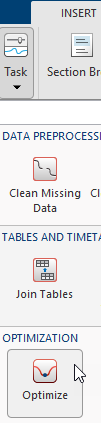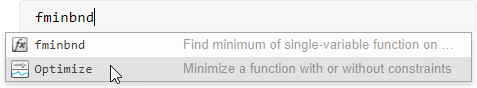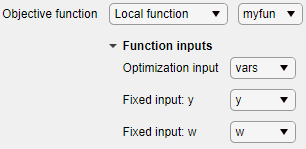# Optimize

Optimize in the Live Editor

## Description

The Optimize task lets you interactively optimize linear and nonlinear objective functions subject to constraints of various types, and to solve nonlinear systems of equations. The task automatically generates MATLAB® code for your live script.

• Specify the objective function, either by writing a function or browsing for a function.

• Specify solver options.

• Run the optimization.

To add the Optimize task to a live script in the MATLAB Editor, on the Live Editor Insert tab, select Task > Optimize.Alternatively, in a code block in the script, type a relevant keyword, such as `fminbnd`. Select Optimize from the suggested command completions.## Parameters

Optimization solver that MATLAB uses to solve the problem, specified as one of these solvers:

Example: `fminsearch`

Function to minimize (for `fminbnd` or `fminsearch`) or to find a root (for `fzero`), specified as a function handle, local function name, or function file.

• Function handle — Create the function handle in the workspace. Then, in the Optimize task, select Objective function > Function handle and choose the objective function.

• Local function

• New — In the Optimize task, select Objective function > Local function and then click the button. A new function definition appears below the task. Edit the function so that it calculates the objective. Then choose the local function.

• Existing — Select Objective function > Local function and choose the local function.

• Function file

• New — In the Optimize task, select Objective function > From file and then click the button. A new function file appears. Edit the file to calculate the objective, then save the file on the MATLAB path. Click the button and select the file.

• Existing — Choose `From file` then Browse... and select the file.

## Tips

• For functions with extra inputs, Optimize requires you to choose the optimization variable, and to specify which workspace variables contain the fixed data inputs. This example contains three function inputs:Optimize generates code only after you specify all function inputs.

• Optimize cannot parse a function containing the `varargin` input or a function that contains an error.

• If you select an objective function from a file, Optimize adds the file location to your MATLAB path.

• If Optimize has a parsing error or if multiple local functions have the same name, the list of available local functions is empty.